How Cheenta works to ensure student success?
Explore the Back-Story

# Test of Mathematics Solution Subjective 155 -The Lim 1/(n+r) Problem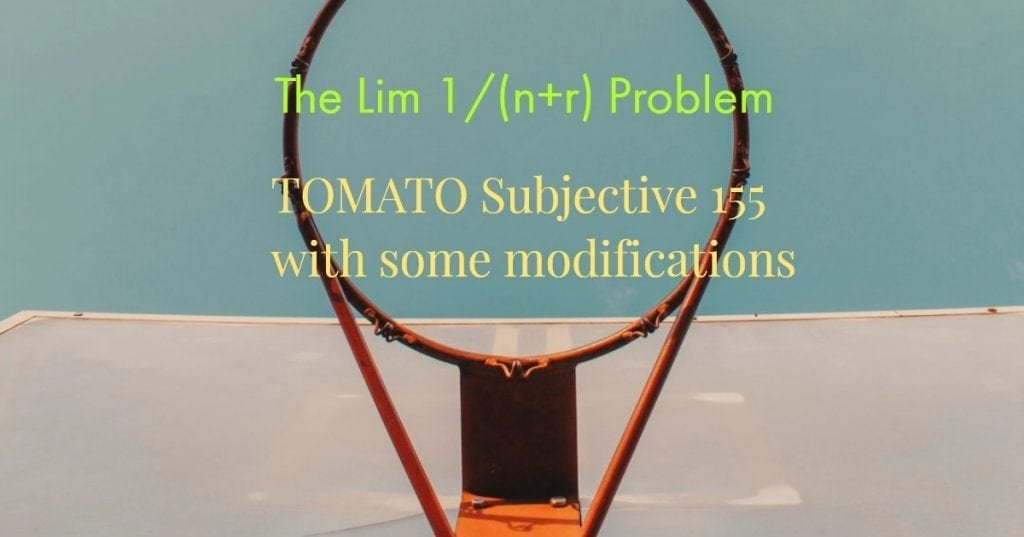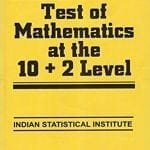This is a Test of Mathematics Solution Subjective 155 (from ISI Entrance). The book, Test of Mathematics at 10+2 Level is Published by East West Press. This problem book is indispensable for the preparation of I.S.I. B.Stat and B.Math Entrance.

Also visit: I.S.I. & C.M.I. Entrance Course of Cheenta

## Problem

Evaluate: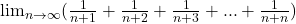## Solution

As the title suggests the modification of this problem will be, that we will solve a more general series and then use a specific value to arrive at the solution of this problem.

First let us consider the following limit:Observe carefully that using k=1 in this limit, we get the limit that has been asked to evaluate.

Now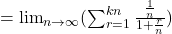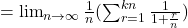Let's substitute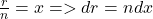Now we can change the sum to an integral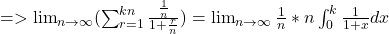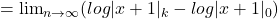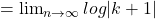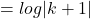(As the term is an 'n' free term)

So we see the solution isSubstituting k=1, we get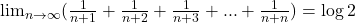This is a Test of Mathematics Solution Subjective 155 (from ISI Entrance). The book, Test of Mathematics at 10+2 Level is Published by East West Press. This problem book is indispensable for the preparation of I.S.I. B.Stat and B.Math Entrance.

Also visit: I.S.I. & C.M.I. Entrance Course of Cheenta

## Problem

Evaluate:## Solution

As the title suggests the modification of this problem will be, that we will solve a more general series and then use a specific value to arrive at the solution of this problem.

First let us consider the following limit:Observe carefully that using k=1 in this limit, we get the limit that has been asked to evaluate.

NowLet's substituteNow we can change the sum to an integral(As the term is an 'n' free term)

So we see the solution isSubstituting k=1, we get### Knowledge Partner